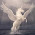### Coursera: Quantum Mechanics and Quantum Computation

Quantum Mechanics and Quantum Computation

Quantum computation is a remarkable subject, and is based on one of the great computational discoveries that computers based on quantum mechanics are exponentially powerful. This course aims to make this cutting-edge material broadly accessible to undergraduate students, including computer science majors who do not have any prior exposure to quantum mechanics. The course will introduce qubits (or quantum bits) and quantum gates, the basic building blocks of quantum computers. It will cover the fundamentals of quantum algorithms, including the quantum fourier transform, period finding, and Shor's iconic quantum algorithm for factoring integers efficiently. The course will also explore the prospects for quantum algorithms for NP-complete problems and basic quantum cryptography.

The course will not assume any prior background in quantum mechanics. Instead, it will use the language of qubits and quantum gates to introduce the basic axioms of quantum mechanics. This treatment of quantum mechanics has the advantage of both being conceptually simple and of highlighting the paradoxes at the heart of quantum mechanics. The most important pre-requisite for the course is a good understanding of basic linear algebra, including vectors, matrices, inner products, eigenvectors and eigenvalues, etc.Umesh Vazirani is the Strauch Distinguished Professor of Electrical Engineering and Computer Science at University of California, Berkeley, and is the director of the Berkeley Quantum Information and Computation Center. Prof. Vazirani has done foundational work on the computational foundations of randomness, algorithms and novel models of computation. His 1993 paper with Ethan Bernstein helped launch the field of quantum complexity theory. He is the author of two books "An introduction to computational learning theory" with Michael Kearns and "Algorithms" with Sanjoy Dasgupta and Christos Papadimitriou.

1.thanks for sharing.

2.Hi, I can't get the videos of this course, for I was not signed in :(

It says to me "We are sorry, but the enrollment for Quantum Mechanics and Quantum Computation is currently closed". Where can I get the video lectures now? :(

1.I do believe they were available for download during the course, so someone may have made them available as a BitTorrent.

### A Bayes' Solution to Monty Hall

For any problem involving conditional probabilities one of your greatest allies is Bayes' Theorem. Bayes' Theorem says that for two events A and B, the probability of A given B is related to the probability of B given A in a specific way.

Standard notation:

probability of A given B is written $$\Pr(A \mid B)$$
probability of B is written $$\Pr(B)$$

Bayes' Theorem:

Using the notation above, Bayes' Theorem can be written: $\Pr(A \mid B) = \frac{\Pr(B \mid A)\times \Pr(A)}{\Pr(B)}$Let's apply Bayes' Theorem to the Monty Hall problem. If you recall, we're told that behind three doors there are two goats and one car, all randomly placed. We initially choose a door, and then Monty, who knows what's behind the doors, always shows us a goat behind one of the remaining doors. He can always do this as there are two goats; if we chose the car initially, Monty picks one of the two doors with a goat behind it at random.

Assume we pick Door 1 and then Monty sho…

### Mixed Models in R - Bigger, Faster, Stronger

When you start doing more advanced sports analytics you'll eventually starting working with what are known as hierarchical, nested or mixed effects models. These are models that contain both fixed and random effects. There are multiple ways of defining fixed vs random random effects, but one way I find particularly useful is that random effects are being "predicted" rather than "estimated", and this in turn involves some "shrinkage" towards the mean.

Here's some R code for NCAA ice hockey power rankings using a nested Poisson model (which can be found in my hockey GitHub repository):
model <- gs ~ year+field+d_div+o_div+game_length+(1|offense)+(1|defense)+(1|game_id) fit <- glmer(model, data=g, verbose=TRUE, family=poisson(link=log) ) The fixed effects are year, field (home/away/neutral), d_div (NCAA division of the defense), o_div (NCAA division of the offense) and game_length (number of overtime periods); off…

### Gambling to Optimize Expected Median Bankroll

Gambling to optimize your expected bankroll mean is extremely risky, as you wager your entire bankroll for any favorable gamble, making ruin almost inevitable. But what if, instead, we gambled not to maximize the expected bankroll mean, but the expected bankroll median?

Let the probability of winning a favorable bet be $$p$$, and the net odds be $$b$$. That is, if we wager $$1$$ unit and win, we get back $$b$$ units (in addition to our wager). Assume our betting strategy is to wager some fraction $$f$$ of our bankroll, hence $$0 \leq f \leq 1$$. By our assumption, our betting strategy is invariant with respect to the actual size of our bankroll, and so if we were to repeat this gamble $$n$$ times with the same $$p$$ and $$b$$, the strategy wouldn't change. It follows we may assume an initial bankroll of size $$1$$.

Let $$q = 1-p$$. Now, after $$n$$  such gambles our bankroll would have a binomial distribution with probability mass function \[ \Pr(k,n,p) = \binom{n}{k} p^k q^{n-k…